Transcripted Summary

The last decision structure we'll look at is the switch statement.

The Switch Statement

If situation A occurs, do something.

Else if situation B occurs, do something else.

Else if situation C occurs, do something else.

The switch statement solves a problem in the same way that the if-else-if does. So, it's ideal for cases when you have more than two paths. The difference between the if-else-if and the switch statements is that the if-else-if checks the condition to be true; whereas the switch statement checks for equality.

Let's look at an example.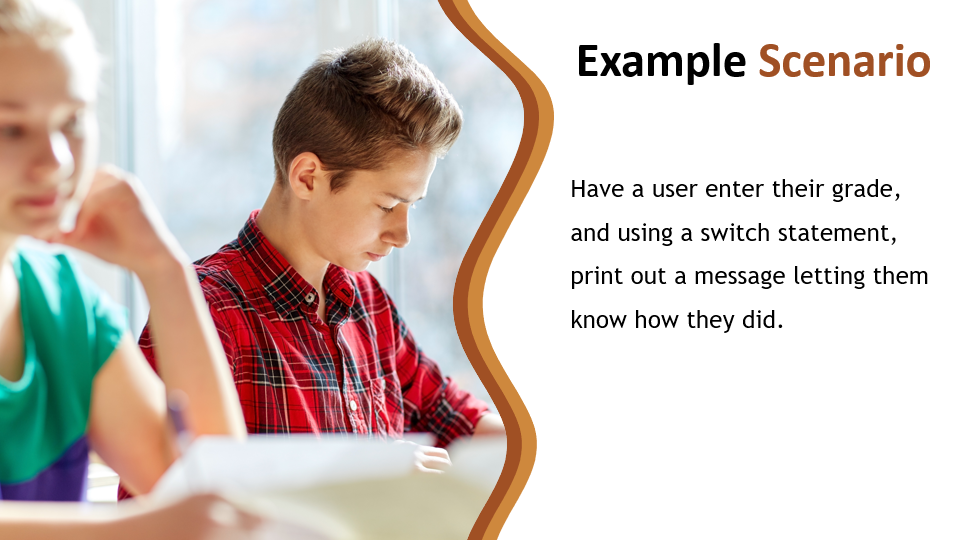In the last section we used if-else-if because we had a large range of scores to cover, 0 to 100, in fact.

But, in this example, it's asking us to simply print a message based on the student's letter grade. There are only 5 possible values in this case, so checking for equality is not so daunting.

Let's code this up.

I'll make a new class in chapter3 packaged called `GradeMessage`

``````/*
* SWITCH
* Have a user enter their letter grade, and using a switch statement,
* print out a message letting them know how they did.
*/

}
``````

Let's go ahead and get the letter grade from the user within the main method.

``````public class GradeMessage {

public static void main(String args[]){
Scanner scanner = new Scanner(System.in);
}
}
``````

Now the letter grade that we're going to read in is essentially going to be one letter. So, it's going to be a character however the `Scanner` does not have a method to get just a character. The closest we have is a String which is also used for text. Even though it's going to be only one letter we can still hold it in a String variable.

So, let's use `next` as there is no `nextString`. The `next` method by default, we see that it returns to String.

And let's store this in a String data type.

``````String grade = scanner.next();
Scanner.close();
``````

Okay, now we're ready for our switch decision structure.

In order to invoke this, we type `switch` and then inside parentheses we give it the variable that we want it to evaluate so we want it to evaluate `grade` and based on this we'll determine the path that we take.

``````switch(grade){

}
``````

Inside of the `switch` curly braces, we enter a number of cases, and each case is a path. So, we can say that in the case that grade equals A, then we put a colon and anything that we want to happen.

``````switch(grade){
case "A":
}
``````

We can state that in the case of grade equalling “A”, we want the message to be “Excellent job!”.

I'm going to go ahead and make a variable to hold this String, and we don't know what it is yet, so we won't initialize it.

But inside of this first case, we'll go ahead and update `message` with the value.

``````String message;

case "A":
message = "Excellent job!";
break;
}
``````

You end your `case` with this `break` reserved word. And that closes out this `case`.

Formatting for Switch Statements

We don't use the curly bases for the cases, instead we use this colon to begin. We can add however many statements we would like and when we're done with that case, we add the `break`.

Now I'm ready for the next cases.

``````switch(grade){
case "A":
message = "Excellent job!";
break;
case "B":
message = "Great job!";
break;
case "C":
message = "Good job!";
break;
case "D":
message = "You need to work a bit harder";
break;
case "F":
message = "Uh oh!";
break;
}
``````

Okay. So that covers the five cases.

But what if they answer something different than this? Some type of error, or whatever. If we don't have a case for it then nothing will occur. So, in the `switch` statement, it's a good practice to also have a `default` case that handles anything else.

The default case is denoted by the word `default` then the colon; and then the same thing you do with the cases.

``````default:
break;
``````

So that is the `switch` statement.

Notice here that it's kind of similar in the way that it processes just like the if-else-if, right? So we could've easily implemented this with if-else-if statements. We could've said: If the grade equals A, then do this. If the grade equals B, yada, yada, yada.

So, these are interchangeable in that way.

The other thing is, I said earlier how the if-else-if dealt with conditions, so let’s look at that class again.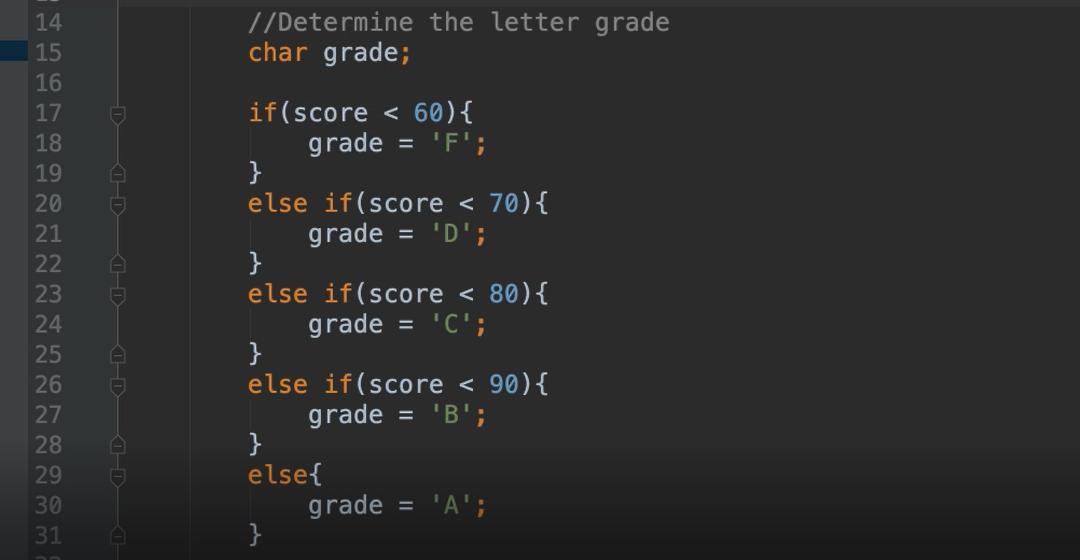These conditions are using the less than operator to cover more than one value. So, this allows us to have a nice range of values.

This `switch` statement works differently. It is not comparing the condition in the same way; it is comparing a certain condition — equality. So, in the TestResults class, we could've put anything we wanted to: <, >, >=, anything. But in the case of a `switch`, the condition is equality.

Let’s review a case again.

`````` switch(grade){
case "A":
}
``````

So, `case “A”:` means “does the `grade` equal to A”? If this is true, then this is executed. Then we see alternate options for if the grade equals B, C, D, or F.

Just like the if-else-if, only one of these will be invoked — the first one that is true. So, if the grade is equal to A, then case A will be executed. Once this is done, it will not even evaluate the rest of these cases. It will go on to the rest of the program after the switch statement. Same for the if-else-if.

Let's say that we had some cases where multiple conditions evaluated to true. Only the first one that is evaluated to be true will be executed. It would never look at the rest of the cases, because these are essentially elses. If this is not true, then consider these. If it finds one of the cases to be true, it will not evaluate the following ones.

However, the switch statement allows what’s called a fall through.

Fall throughs occur when you eliminate the `break` statement at the end of a case.

``````switch(grade){
case "A":
message = "Excellent job!";
case "B":
message = "Great job!";
break;
}
``````

Let’s say the value of `grade` was A. It would execute case A, however, because case A does not have a break statement, it would continue on to execute case B, even though `grade` doesn’t even equal B! In fact, it will keep going through all the cases until it reaches a break statement.

Now sometimes that's by design; but most times it's because someone forgot to put a `break`. So, you have to be very careful when you use the `switch` statement to make sure you have this `break` keyword when you want it to stop.

So, let's go ahead and output our message.

``````System.out.println(message);
``````

Now I'm going to go ahead and show you this with and without the fall through, so let's run this.

``````package chapter3;

import java.util.Scanner;

/*
* SWITCH
* Have a user enter their letter grade, and using a switch statement,
* print out a message letting them know how they did.
*/

public static void main(String args[]){

Scanner scanner = new Scanner(System.in);
scanner.close();

String message;

case "A":
message = "Excellent job!";
break;
case "B":
message = "Great job!";
break;
case "C":
message = "Good job!";
break;
case "D":
message = "You need to work a bit harder";
break;
case "F":
message = "Uh oh!";
break;
default:
break;
}

System.out.println(message);

}
}
``````

We enter “C” as the grade and we see that it evaluated case A which was false, case B which was false, then case C which was true, so it prints “Good job!”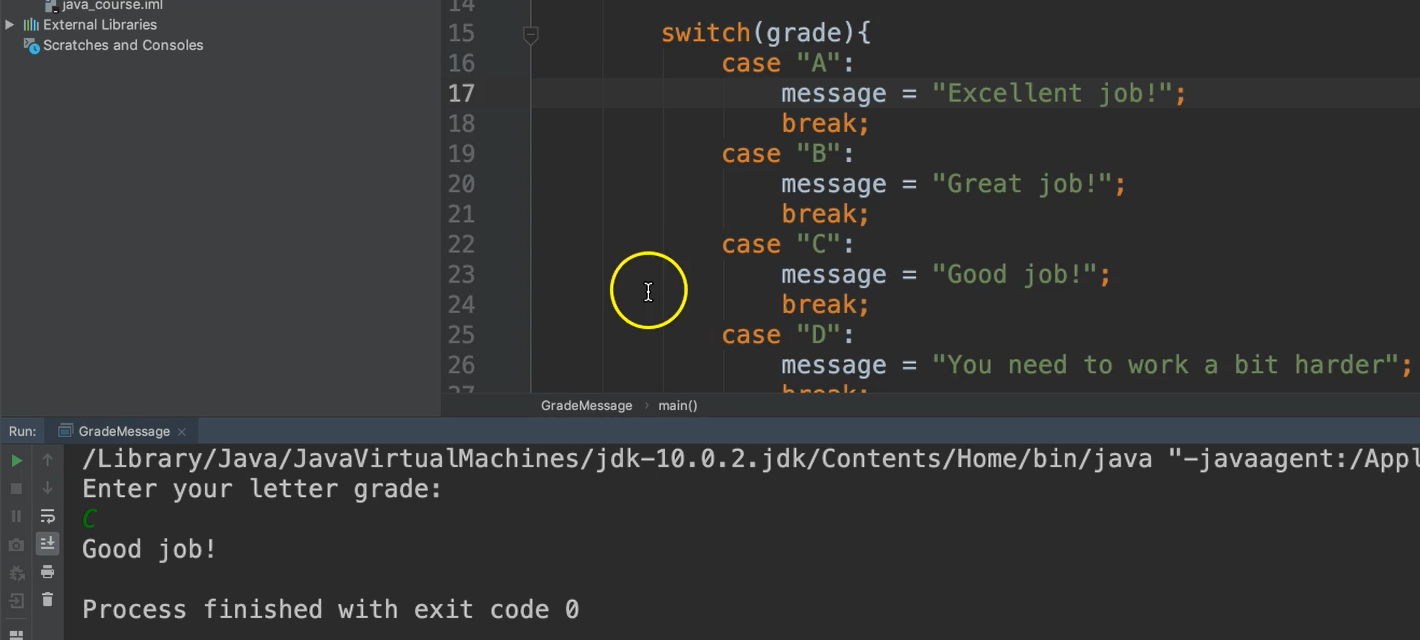Let's remove the break statement from case C.

Now in this case, it's going to do a fall through. So, it's going to keep going even though case D is not met.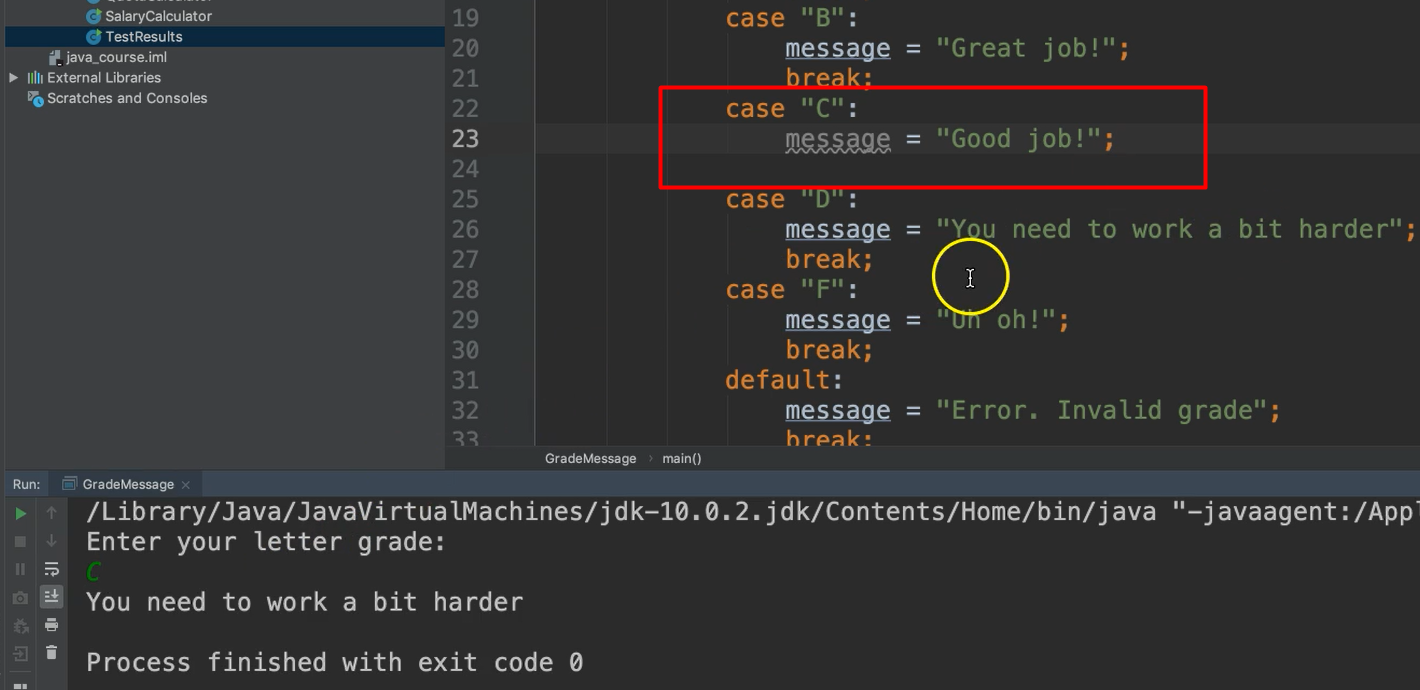We entered “C”, but it printed out the message associated with case D. Why is that?

We see that the message was set as “Good job!” in case C, that did occur. However, it kept going to case D and it overrode the value that was in the message variable. So by the time we printed out, it had gotten to case D. That's why it's important to have that `break` statement.

Let's go ahead and add it back and let's go for the default case now. I'm going to trick you. I'm going to put the letter c, lowercase.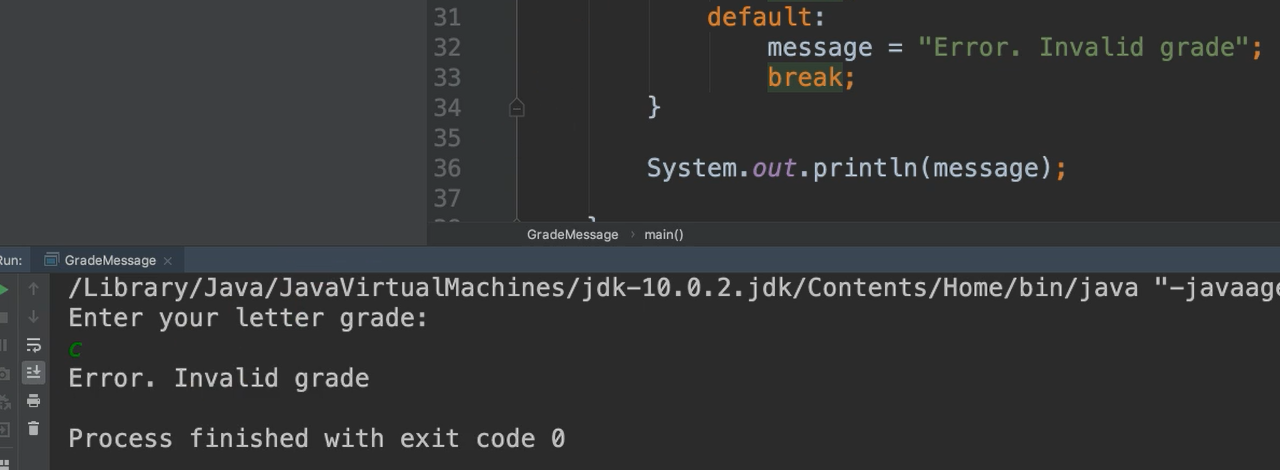So, you would expect it to still go to case C, but this is case sensitive. So, remember, Java is case sensitive.

Most times it's better to use an if-else-if statement because it allows for a range of conditions not just equality. Also, you don't have to remember to put those break statements.

There are some cases where you might want to use the `switch` and that's perfectly fine as well. It's up to you to decide which is best for the problem that you're solving.

Okay. That's the switch statement.